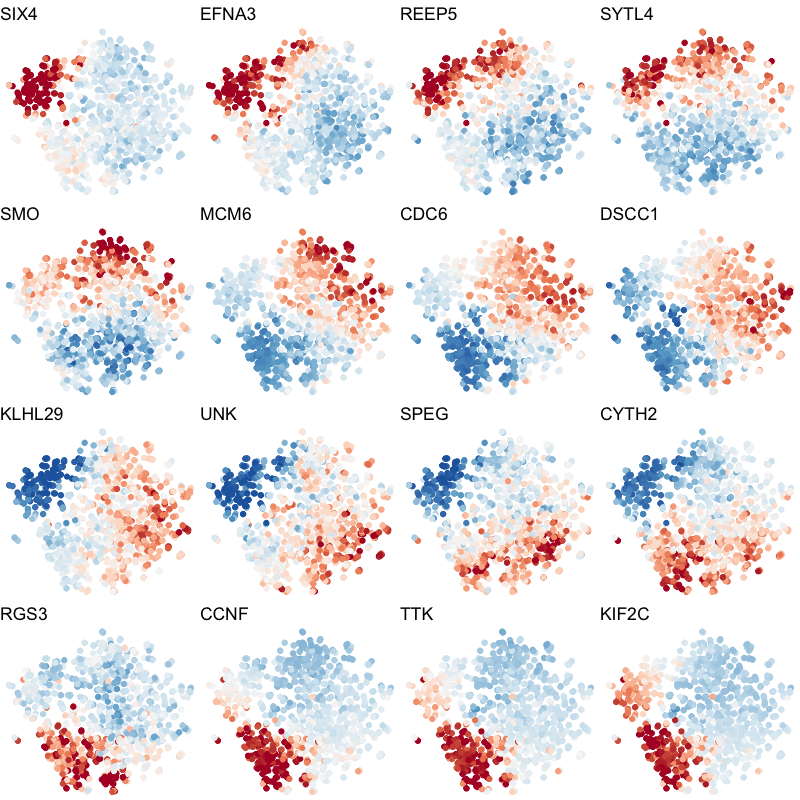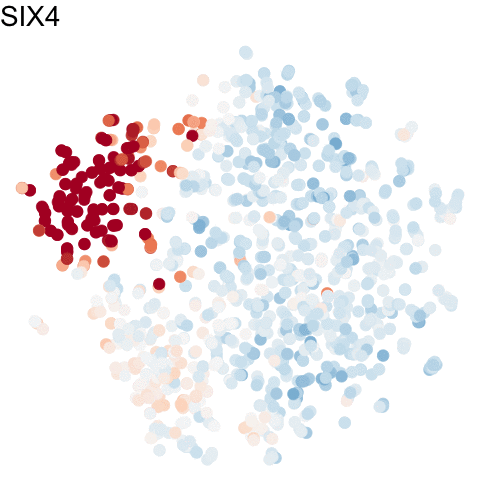# Animating the Cell Cycle

Dec 28, 2020

## Introduction

There are many ways to visualize the same data. In Xia*, Fan*,Emanuel* et al (2019), we used transcriptome-scale MERFISH along with RNA velocity in situ analysis to identify genes associated with the cell cycle. For more details on the analysis, please refer to the original manuscript or check out RNA Velocity Analysis (In Situ) - Tutorials and Tips.

## Static visualizations

To visualize these identified cell cycle genes, we used a variety of different static visualizations, including those similar to the ones below. These tSNE plots visualize cells as points and are colored by scaled gene expression magnitude of select identified cell cycle genes.

``````m <- mat[genes.final,]
m <- t(scale(t(m)))
m[m > 2] <- 2
m[m < -2] <- -2

library(ggplot2)
ps <- lapply(seq_along(genes.final), function(i) {
gexp <- m[genes.final[i], rownames(emb.test)]
df <- data.frame(emb.test, gexp)
p <- ggplot(df, aes(x = X1, y = X2, col=gexp)) + geom_point()
p <- p + theme_void() +
theme(legend.position = "none") +
scale_color_distiller(palette = 'RdBu',
limits = c(-2,2)) +
labs(title = genes.final[i])
p
})

library(gridExtra)
grid.arrange(
grobs = ps,
nrow=4, ncol=4
)
``````In the original manuscript, we also used heatmaps to visualize the pseudotemporal ordering of cells versus the peak expression of identified cell cycle genes and scatterplots of the pseudotemporal ordering of cells versus gene expression magnitude to visualize the shifting peak expression of cell cycle genes along pseudotime. Each visualization seeks to communicate and highlight a different aspect of the results.

## Animating with `gganimate`

Still, when we are able to operature outside of traditional printed media and static visualizations, we can now take advantage of the additional time dimension for visualization offered by animations! We can use the `gganimate` package to visualize the same cell cycle genes as above.

``````library(gganimate)

df.all <- do.call(rbind, lapply(1:length(genes.final), function(i) {
gexp <- m[genes.final[i], rownames(emb.test)]
df <- data.frame(emb.test, gexp, gene=genes.final[i], order=i)
}))
p <- ggplot(df.all, aes(x = X1, y = X2, col=gexp)) + geom_point()
p <- p + theme_void() +
theme(legend.position = "none") +
scale_color_distiller(palette = 'RdBu',
limits = c(-2,2))
anim <- p +
transition_states(order,
transition_length = 5,
state_length = 1) +
labs(title = '{genes.final[as.integer(closest_state)]}') +
theme(plot.title = element_text(size = 28)) +
geom_point(size = 5) +